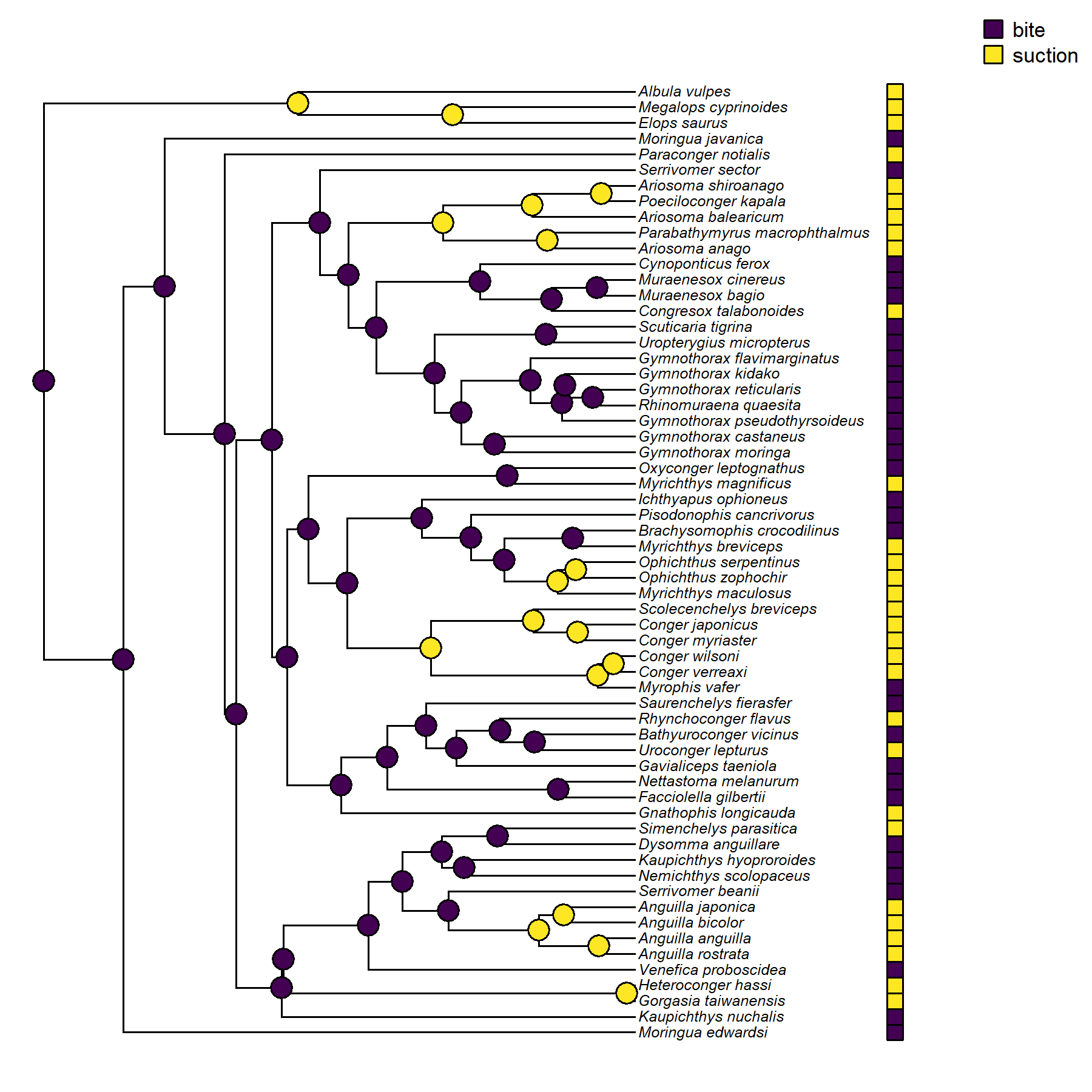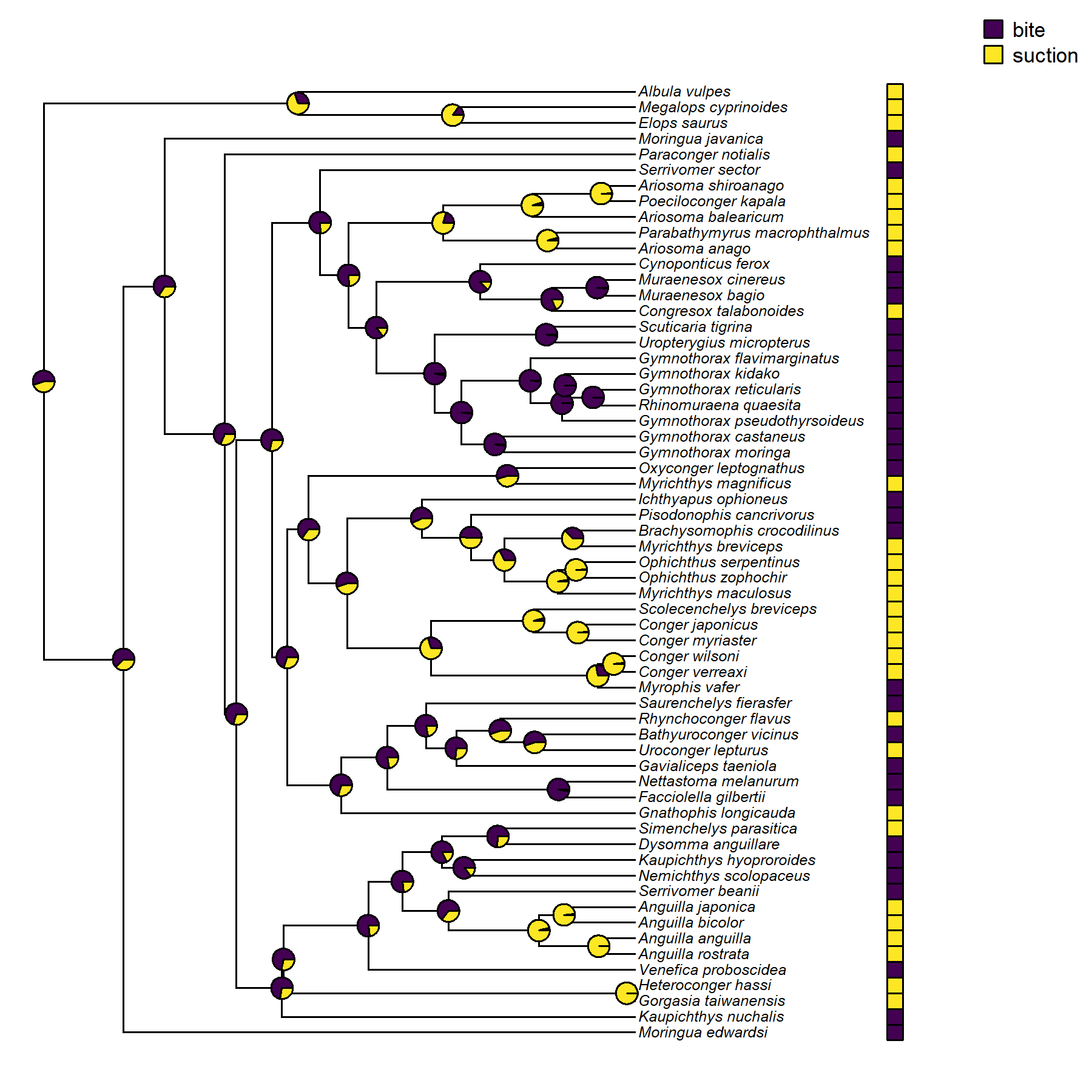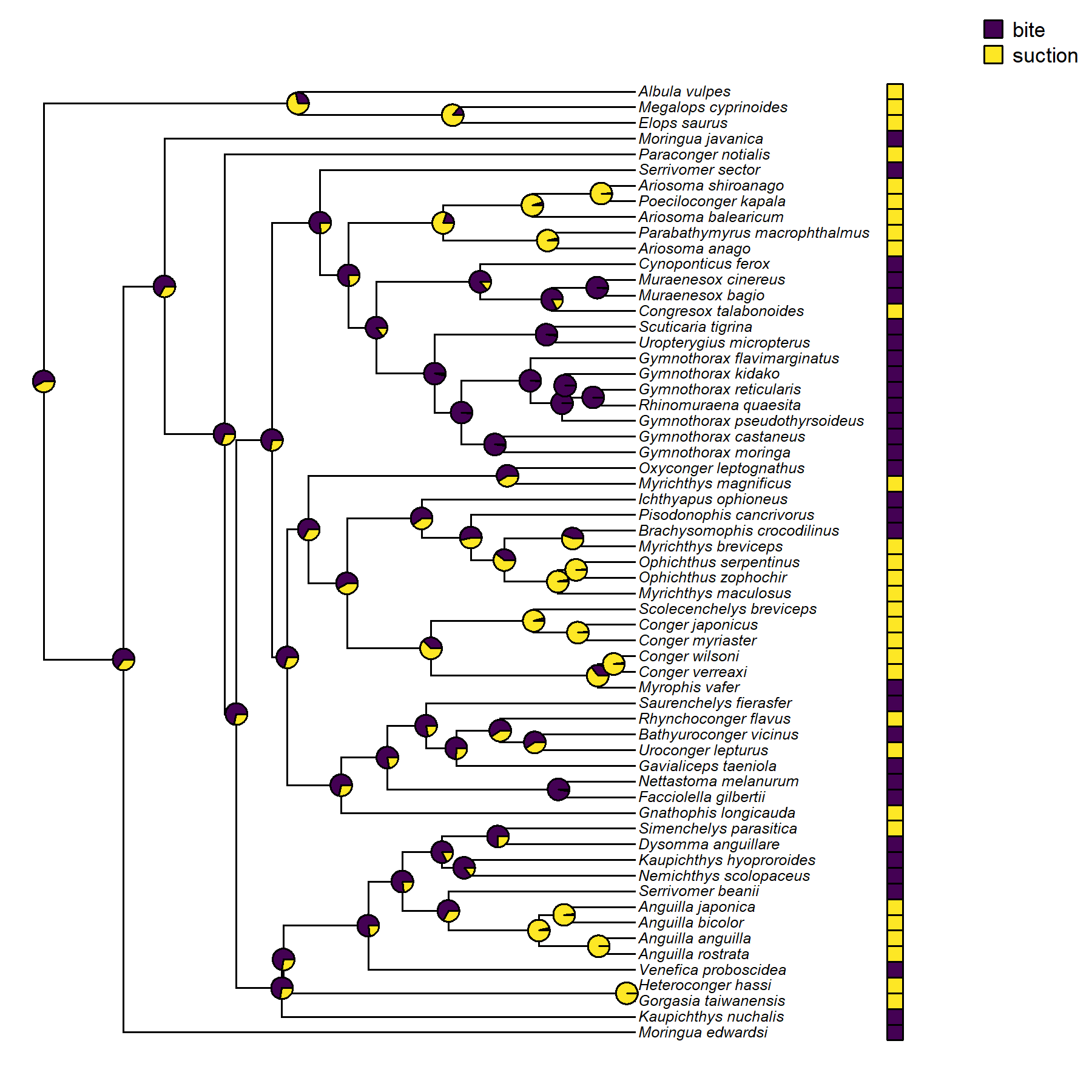### 1. Joint and marginal ancestral state estimation using `phytools::ancr`

In the book we used the excellent R package corHMM for joint and marginal ancestral state estimation of discrete characters. Since that time, I (LJR) have implemented both types of ancestral state reconstruction in phytools in the form of an easy-to-use generic method called `ancr`.

To demonstrate it, we’ll use the same example (feeding mode evolution in elopomorph eels) that appeared in Chapter 8 of our book.

``````library(phytools)
row.names=1,stringsAsFactors=TRUE)``````

Next, we’ll pull out the specific discrete trait that we’re interested in from `eel.data` as a factor vector with names.

``````feed.mode<-setNames(eel.data\$feed_mode,rownames(eel.data))
levels(feed.mode)``````
``##  "bite"    "suction"``

In the book, we saw that the best supported model (accounting for parameter complexity) was the equal-rates (“ER”) model, so let’s just fit that model as we did in Chapter 8.

``````fitER<-fitMk(eel.tree,feed.mode,model="ER")
fitER``````
``````## Object of class "fitMk".
##
## Fitted (or set) value of Q:
##              bite   suction
## bite    -0.015828  0.015828
## suction  0.015828 -0.015828
##
## Fitted (or set) value of pi:
##    bite suction
##     0.5     0.5
## due to treating the root prior as (a) flat.
##
## Log-likelihood: -37.033072
##
## Optimization method used was "nlminb"``````

Rather than re-fit our model, as we did using corHMM, to obtain joint ancestral states, we simply pass this fitted model object to `ancr` along with the argument value `type="joint"`. (Note that corHMM has stand-alone function for ancestral state estimation that works in a similar way. It’s called `ancRECON`.)

``````fit.joint<-ancr(fitER,type="joint")
fit.joint``````
``````## Joint ancestral state estimates:
##    state
## 62  bite
## 63  bite
## 64  bite
## 65  bite
## 66  bite
## 67  bite
## ...
##
## Log-likelihood = -43.086588``````

Now, let’s proceed to plot these results on the tree, just as we did in Chapter 8 of the book. For this code chunk we’re also using the R color palette package viridisLite. (We didn’t use this palette in the book – though we probably should have!)

``````cols<-setNames(viridisLite::viridis(n=2),levels(feed.mode))
plotTree.datamatrix(eel.tree,as.data.frame(feed.mode),
legend("topright",legend=levels(feed.mode),pch=22,
pt.cex=1.5,pt.bg=cols,bty="n",cex=0.7)
nodelabels(pie=to.matrix(fit.joint\$ace,levels(feed.mode)),
piecol=cols,cex=0.5)``````Joint ancestral state reconstruction of feeding mode on the phylogeny of elopomorph eels obtained using the phytools function `ancr`. Compare to Figure 8.9 of the book.

Now, let’s do the same, but this time setting `type="marginal"`. This will give us the marginal reconstruction.

``````fit.marginal<-ancr(fitER,type="marginal")
fit.marginal``````
``````## Marginal ancestral state estimates:
##        bite  suction
## 62 0.548004 0.451996
## 63 0.624234 0.375766
## 64 0.656090 0.343910
## 65 0.690414 0.309586
## 66 0.706649 0.293351
## 67 0.715810 0.284190
## ...
##
## Log-likelihood = -37.033072``````
``````cols<-setNames(viridisLite::viridis(n=2),levels(feed.mode))
plotTree.datamatrix(eel.tree,as.data.frame(feed.mode),
legend("topright",legend=levels(feed.mode),pch=22,
pt.cex=1.5,pt.bg=cols,bty="n",cex=0.7)
nodelabels(pie=fit.marginal\$ace,piecol=cols,cex=0.5)``````Marginal ancestral state reconstruction of feeding mode on the phylogeny of elopomorph eels obtained using the phytools function `ancr`. Compare to Figure 8.10 of the book.

Great!

## 2. Model-averaged ancestral states using `ancr`

The phytools `ancr` function offers a few different “advanced” features not available in other R software for ancestral state estimation.

One of these is a simplified workflow for model-averaging. Here we’ll compute (marginal) ancestral states under each model in a set, but then integrate across models using the Akaike weight of evidence in support of each model.

To take advantage of this workflow, we need to start by fitting the set of models that we’d like to average. In this case, I’ll add the all-rates-different model (“ARD”), as well as the two different directional evolution model of our binary trait. This is the same set of four models that we tested in Chapter 8 of our book. Although this is the comprehensive set of extended Mk models for a binary trait, for characters with a bigger state space, we may want to be somewhat more selective about the selection of models we include in our set.

``````fitARD<-fitMk(eel.tree,feed.mode,model="ARD")
fit01<-fitMk(eel.tree,feed.mode,model=matrix(c(0,1,0,0),2,2,
byrow=TRUE))
fit10<-fitMk(eel.tree,feed.mode,model=matrix(c(0,0,1,0),2,2,
byrow=TRUE))``````

Having fit them, we should next compare our four models using a generic `anova` function call as follows. Here we need to be careful to save our results to a new object: in this case, we’ve called this object `aov_fits`.

``aov_fits<-anova(fitER,fit01,fit10,fitARD)  ``
``````##           log(L) d.f.      AIC     weight
## fitER  -37.03307    1 76.06614 0.62095533
## fit01  -38.64057    1 79.28114 0.12443204
## fit10  -40.50138    1 83.00276 0.01935504
## fitARD -37.00365    2 78.00730 0.23525758``````

Now all that we need to do is pass our `aov_fits` object to `ancr` as follows. This will give us our marginal ancestral states in which the scaled likelihoods at each node have been weighted by the Akaike weights of each model in our set.

``````fit.weighted<-ancr(aov_fits)
fit.weighted``````
``````## Marginal ancestral state estimates:
##        bite  suction
## 62 0.585371 0.414629
## 63 0.648491 0.351509
## 64 0.673999 0.326001
## 65 0.699948 0.300052
## 66 0.712925 0.287075
## 67 0.722864 0.277136
## ...
##
## Log-likelihood = -37.068403``````

Lastly, let’s graph our weighted marginal reconstruction on the tree.

``````cols<-setNames(viridisLite::viridis(n=2),levels(feed.mode))
plotTree.datamatrix(eel.tree,as.data.frame(feed.mode),
legend("topright",legend=levels(feed.mode),pch=22,
pt.cex=1.5,pt.bg=cols,bty="n",cex=0.7)
nodelabels(pie=fit.weighted\$ace,piecol=cols,cex=0.5)``````Weighted marginal ancestral state reconstruction of feeding mode on the pylogeny of elopomorph eels.

## 3. Generic `plot` method for `"ancr"` object class

In addition to model averaging, `ancr` also has various custom generic methods, including a `plot` method. To illustrate this method we’ll use the R pipe (`%>%`), but that’s not necessary of course. This can sometimes greatly simplify our workflow in obtaining a visualization of the reconstructed ancestral states on our phylogeny.

``````library(dplyr)
par(mfrow=c(1,2))
ancr(fitER,type="joint")%>%plot(
args.plotTree=list(fsize=0.5,mar=c(0.1,0.1,2.1,0.1)),
args.nodelabels=list(cex=0.7),args.tiplabels=list(cex=0.5))